# Design

The Design blog is all about product and Qlik solutions, such as scripting, data modeling, visual design, extensions, best practices, and more!

Announcements
Action-Packed Learning Awaits! QlikWorld 2023. April 17 - 20 in Las Vegas: REGISTER NOWEmployee

## Set Analysis in the Aggr function

The Aggr() functions is one of the most advanced functions in the QIX engine, and it is not always easy to use. It does not get easier when you put set analysis expressions in it.

In one of my previous posts (Pitfalls of the Aggr function) I recommended having the set analysis expression in both the inner and the outer aggregation function when using set analysis in the Aggr() function. This is a good rule of thumb, because in most cases doing so will generate the result that you want.

But this is not always correct.

In more complex calculations you often need to use the condition in one place only – sometimes in the inner aggregation, sometimes in the outer. It depends on how the condition is formulated. Then it is important to understand the difference between the two positions.

The evaluation of the Aggr() function is a two-step process: In the first step, an intermediary virtual table is created using the inner aggregation and the dimension of the Aggr(). In the second step, this virtual table is aggregated using the outer aggregation.

For example, say that you want to find the largest order value per year. Then you would need to first calculate the sales value per order, and in a second step find the largest of these values. Hence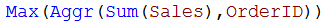The first step aggregates the source data (with multiple records per Order ID) into a virtual table with one record per Order ID, and the second step finds the largest values in the virtual table.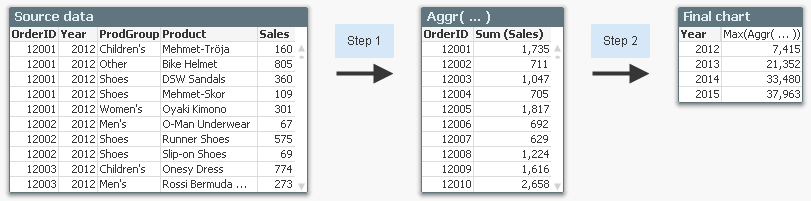However, there is not yet any set analysis in the expression. So, let us use the following requirement instead:

1. Show the largest order value per year
2. Include only products from the product group “Shoes” in the order value
3. Calculate these numbers only for 2014 and 2015

The two conditions correspond to the following set analysis expression: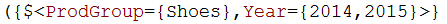But where should this expression be written? In the outer or in the inner aggregation?

To answer this question, we must ask ourselves in which step the conditions should be used. Then it becomes obvious that the condition in product group must be used in step one – in the inner aggregation. If it is used in the outer aggregation only, the order values will be incorrect – they will be calculated from all products.

The condition in year, however, can be put in either place. Hence, the following expression will work fine: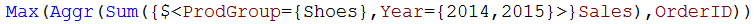From the above example one might draw the conclusion that you always should put the condition in the inner aggregation. But this is not the case. Sometimes you have a condition that cannot be put in the inner aggregation. The following requirement can serve as example:

1. Per year, show the bestselling product within the product group “Shoes”
2. Show how this product ranks compared to all products, also non-shoes

The solution is the following table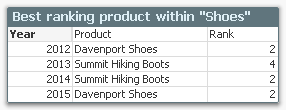The condition in product group should be evaluated in step two, so the expressions used for Product and Rank are:Here it is not possible to have the condition on product group in the inner aggregation, since this would interfere with the calculation of the rank. You must have it in the outer aggregation.

Bottom lines are:

• You need to figure out if your condition should be evaluated in step one (in the inner aggregation) or in step two (in the outer aggregation). This will determine where to put the set analysis expression. You may need to use The Feynman Problem Solving Algorithm.
• If you can’t figure out where to put your set analysis expression – try putting it in both the outer and the inner aggregation function, and keep your fingers crossed. Afterwards you should however verify that the numbers are what you want.

HIC

Further reading related to this topic:

Pitfalls of the Aggr function

A Primer on Set AnalysisMVP

That's interesting, Lucian. Just tried on QV11.20 SR11

=Aggr({1} If(Rank(Sum( AV_Menge))<=20,Only( Sammelartikel)) ,Sammelartikel)

did not work in a list box, I expected the same result like

=Aggr(If(Rank(Sum({1} AV_Menge))<=20,Only({1} Sammelartikel)) ,Sammelartikel)

I would be interested to see an example in QV11.20.

12,193 ViewsSpecialist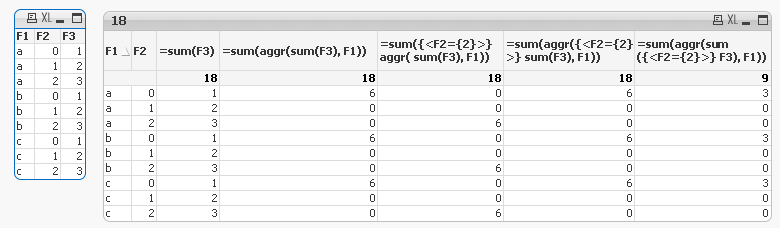This is in QlikView 11.2 SR 8. As you can see, the engine knows the syntax, it calculates it. But the set is applied to the outer result, at least that's how I interpreted the result.

12,193 ViewsMVP

I hope our little discussion is still at least partly on-topic.

Lucian, looking at your sample, it seems to me that the aggr() set analysis isn't considered at all: Isn't the result same as in th 4th column, the advanced aggregation without any set analysis applied?

That's what I've observed in pre QV12 versions, too, the expressions gets evaluated (no calculation error, just a syntax checker error), but the set analysis will just be ignored.

12,018 ViewsSpecialist

Yeah, I didn't give it too much thought... I just applied HIC's rule of thumb: write it everywhere...12,018 Views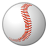Specialist

HI Henric, I was looking out this post and others about Aggr functions, in order to try to solve a problem with table.  Reviewing other post I'm sure that I making a "pitfalls" using that function.

The thing is: Top 5 Customers per ProductType for a given month.  The next expression give me the Rank form this customer

Aggr(Rank(Sum({<FiscalMonth={"\$(=MaxString(FiscalMonth))"}>} Amount)),ProductType,Customer)<=5

But if I try to complicate using this result as an input for a set analysis I don't receive the expected result:

Sum({<Customer={"=Aggr(Rank(Sum({<FiscalMonth={'\$(=MaxString(FiscalMonth))'}>} Amount)),ProductType,Customer)<=5"}>} Amount)

May you check what is my "pitfall".  Thanks

12,018 ViewsMVP

The pitfall is that the advanced search expression should give a single answer for each Customer, TRUE or FALSE.

Basically you are building a virtual table with Customer as dimension and a single expression

=Aggr(Rank(Sum({<FiscalMonth={'\$(=MaxString(FiscalMonth))'}>} Amount)),ProductType,Customer)<=5

And for all kind of expressions, there is a general rule:

Use Aggregation Functions!

The Aggr() is missing the outer aggregation function, so Only() will be applied, and this is not what you want here, right?

12,018 ViewsSpecialist

Thanks swuehl for answer, really that I don't get the solution.  I open a case in order to get some post from the community.

May you check this out? I appreciate your help.

Aggr With Ranking

12,018 Views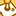Contributor

Hello Henric,

I have created a formula:

The values in SKU level look ok, but when I see the total I needed the average of 76%,100%,100%,100% (...) --> sould be 95% not 100%.

The issue is that the total does the formula itself.

Is there any way to solve this issue? Maybe use AGGR?

tries something like that but did not work at all.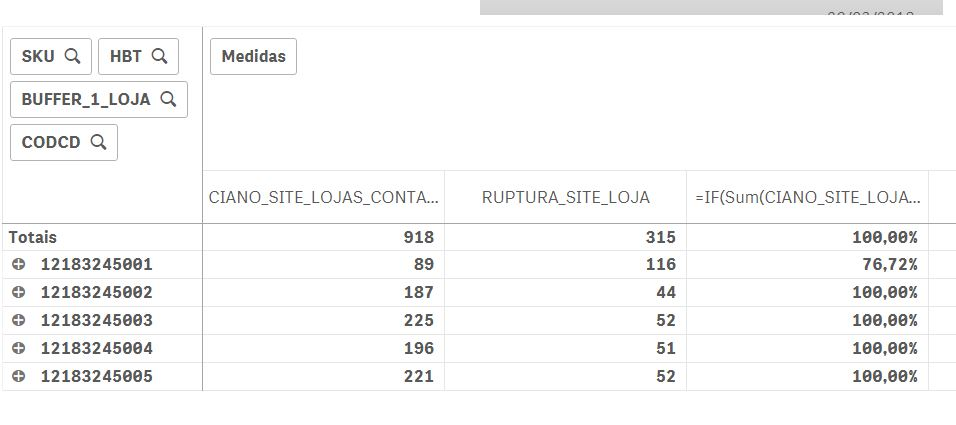12,018 ViewsEmployee

I think you should try the following two alternatives:

1) An unweighted average of the different SKU:s:

Here, the RangeMin() assures that the ratio never is greater than 1.

2) A weighted average of the different SKU:s:

/

Sum(Aggr(Sum(RUPTURA_SITE_LOJA),SKU))

Here the RangeMin() in the numerator assures that the Sum(CIANO_SITE_LOJAS_UNIDADES) never is greater than Sum(RUPTURA_SITE_LOJA).

In both cases, I have used SKU as inner dimension in the Aggr(), but it is likely that you need to replace this by all dimensions of the chart, i.e.

Aggr(..., SKU, HBT, BUFFER_1_LOJA, CODCD)

HIC

12,053 ViewsPartner - Creator II

Hi Henric,

I have an additional question about how set analysis with an aggregation function behaves inside AGGR.

In the following example:

sum(aggr(

count({<Status-={11}, IDZ.For.Count={'\$1'},Date={'>=01.01.2020'}>+<ID_Document={"\$(=max({<Status-={11}, IDZ.For.Count={'\$1'},Date={'<01.01.2020'}>} ID_Document))"}>} IDZ.For.Count)

, ID_Customer))

I have to sum over all customers some document attributes IDZ.For.Count for every individual customer, where this customer's documents are defined depending on their Date - only the ones in year 2020 plus the document with greatest Doc.ID before 2020. This is estimated for every customer, but the second set modifier with Max aggregation is not giving the expected result for each ID_Customer in the aggregation, instead it gives the greatest Doc.ID for the entire selection scope disregarding the aggr function.

Could you please comment on this. Thank you!

3,217 Views
Subscribe by Topic